Скачать презентацию Warm-Up Exercises Name the polygon by the number

498666a26e969543b3cc87b9baa44738.ppt

• Количество слайдов: 22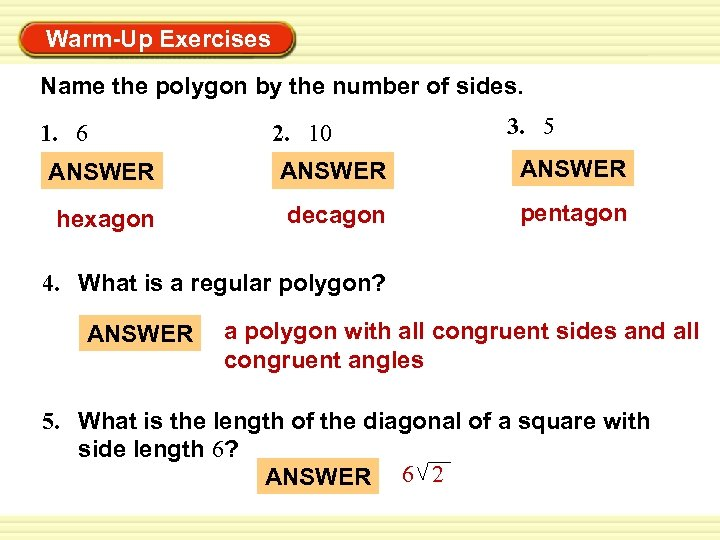Warm-Up Exercises Name the polygon by the number of sides. 1. 6 2. 10 3. 5 ANSWER hexagon decagon pentagon 4. What is a regular polygon? ANSWER a polygon with all congruent sides and all congruent angles 5. What is the length of the diagonal of a square with side length 6? ANSWER 6 2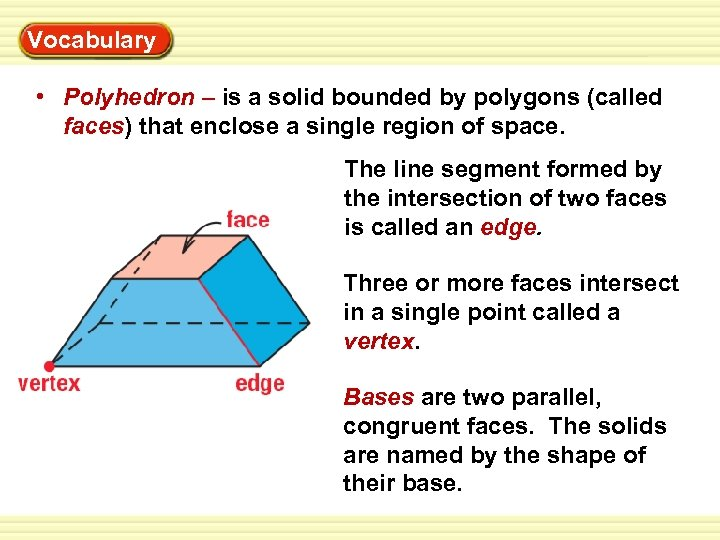Warm-Up Vocabulary Exercises • Polyhedron – is a solid bounded by polygons (called faces) that enclose a single region of space. The line segment formed by the intersection of two faces is called an edge. Three or more faces intersect in a single point called a vertex. Bases are two parallel, congruent faces. The solids are named by the shape of their base.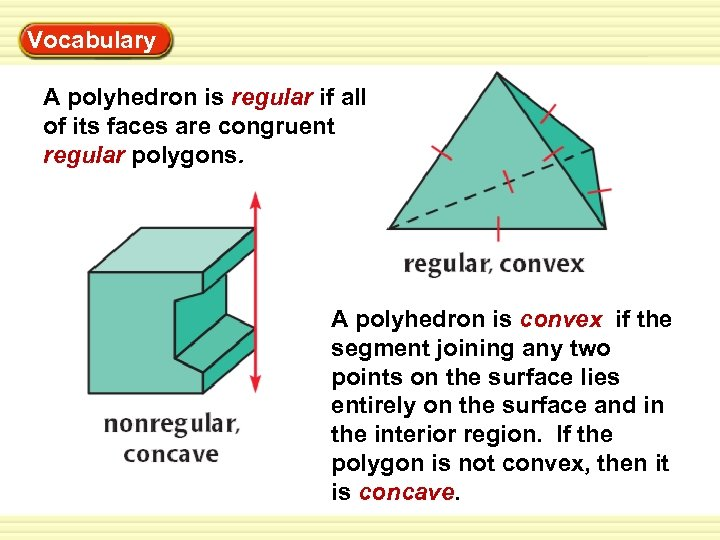Warm-Up Vocabulary Exercises A polyhedron is regular if all of its faces are congruent regular polygons. A polyhedron is convex if the segment joining any two points on the surface lies entirely on the surface and in the interior region. If the polygon is not convex, then it is concave.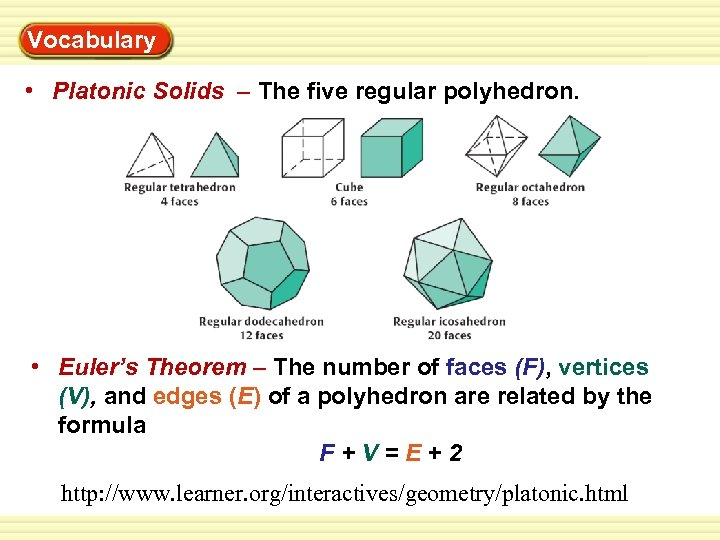Warm-Up Vocabulary Exercises • Platonic Solids – The five regular polyhedron. • Euler’s Theorem – The number of faces (F), vertices (V), and edges (E) of a polyhedron are related by the formula F+V=E+2 http: //www. learner. org/interactives/geometry/platonic. html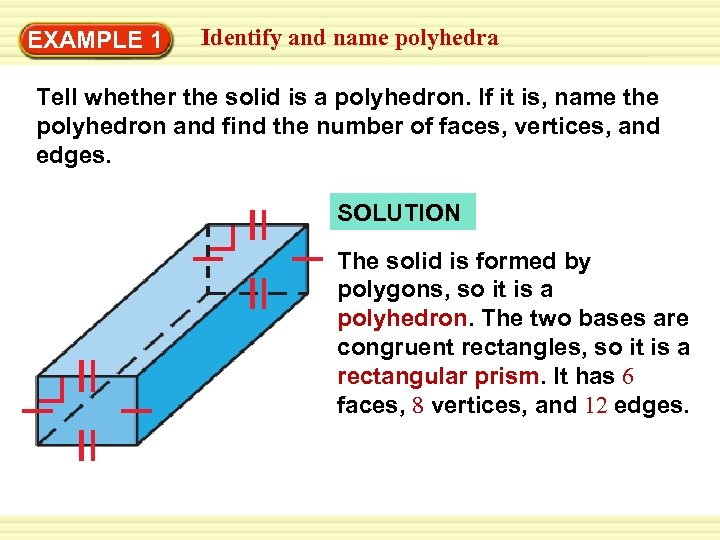Identify Warm-Up EXAMPLE 1 Exercises and name polyhedra Tell whether the solid is a polyhedron. If it is, name the polyhedron and find the number of faces, vertices, and edges. SOLUTION The solid is formed by polygons, so it is a polyhedron. The two bases are congruent rectangles, so it is a rectangular prism. It has 6 faces, 8 vertices, and 12 edges.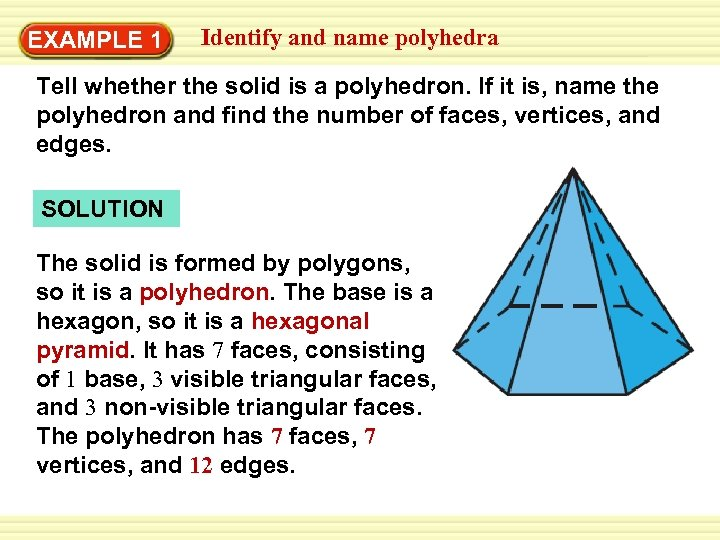Identify Warm-Up EXAMPLE 1 Exercises and name polyhedra Tell whether the solid is a polyhedron. If it is, name the polyhedron and find the number of faces, vertices, and edges. SOLUTION The solid is formed by polygons, so it is a polyhedron. The base is a hexagon, so it is a hexagonal pyramid. It has 7 faces, consisting of 1 base, 3 visible triangular faces, and 3 non-visible triangular faces. The polyhedron has 7 faces, 7 vertices, and 12 edges.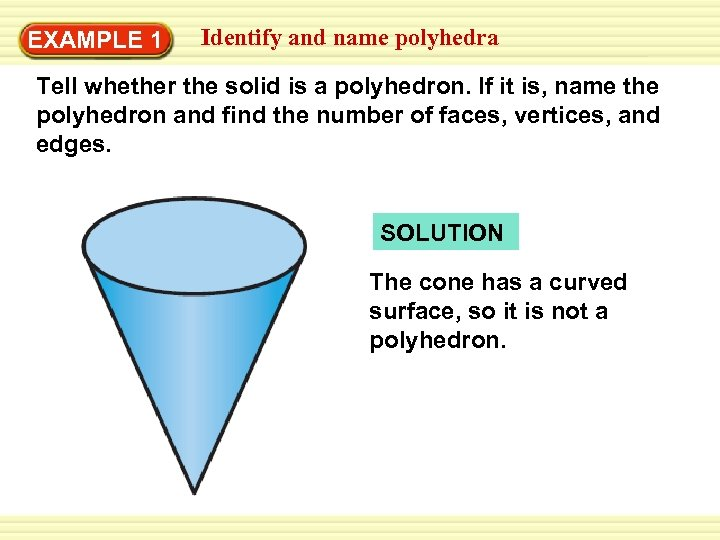Identify Warm-Up EXAMPLE 1 Exercises and name polyhedra Tell whether the solid is a polyhedron. If it is, name the polyhedron and find the number of faces, vertices, and edges. SOLUTION The cone has a curved surface, so it is not a polyhedron.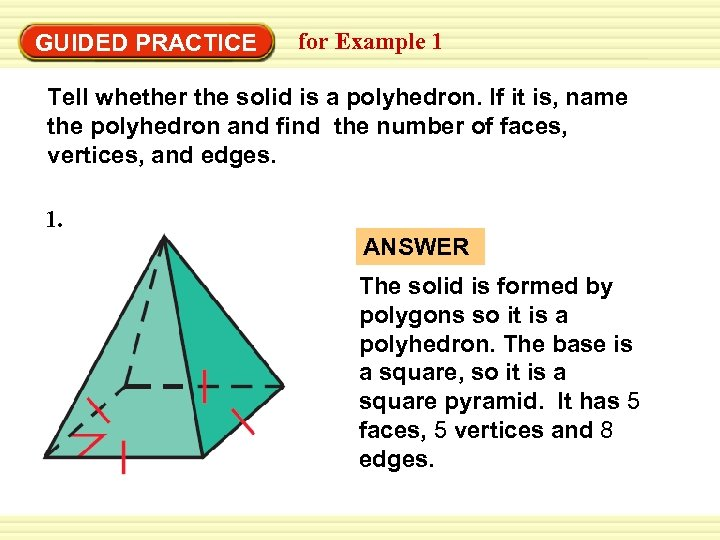Warm-Up Exercises GUIDED PRACTICE for Example 1 Tell whether the solid is a polyhedron. If it is, name the polyhedron and find the number of faces, vertices, and edges. 1. ANSWER The solid is formed by polygons so it is a polyhedron. The base is a square, so it is a square pyramid. It has 5 faces, 5 vertices and 8 edges.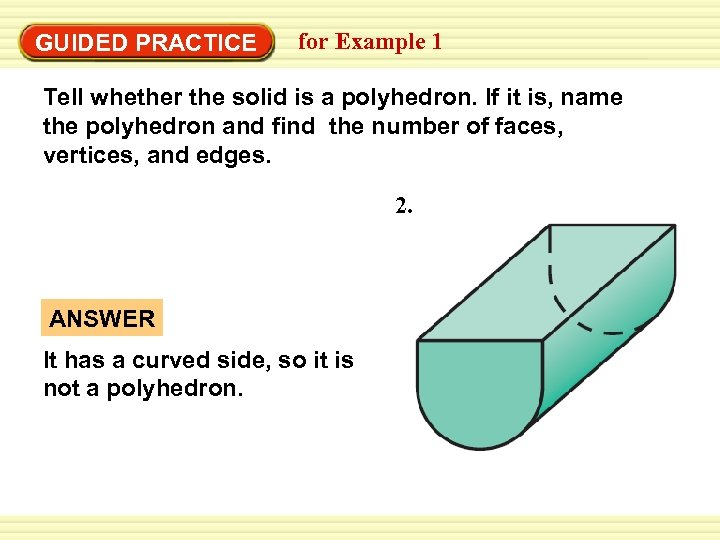Warm-Up Exercises GUIDED PRACTICE for Example 1 Tell whether the solid is a polyhedron. If it is, name the polyhedron and find the number of faces, vertices, and edges. 2. ANSWER It has a curved side, so it is not a polyhedron.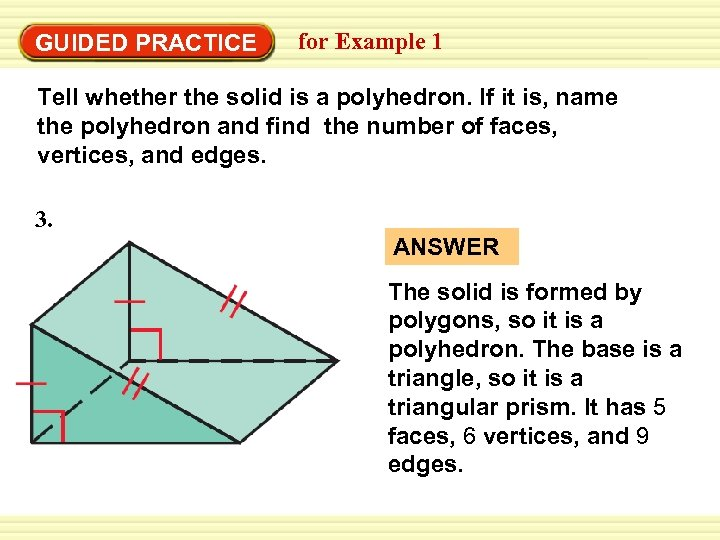Warm-Up Exercises GUIDED PRACTICE for Example 1 Tell whether the solid is a polyhedron. If it is, name the polyhedron and find the number of faces, vertices, and edges. 3. ANSWER The solid is formed by polygons, so it is a polyhedron. The base is a triangle, so it is a triangular prism. It has 5 faces, 6 vertices, and 9 edges.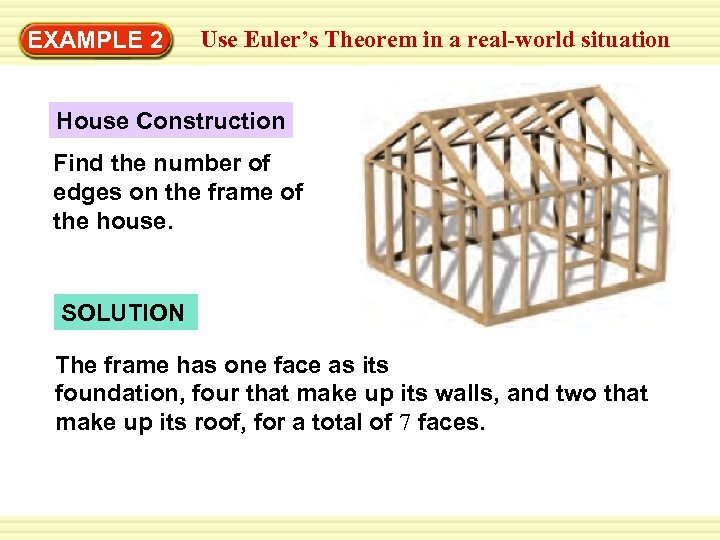Use Euler’s Theorem in a real-world situation Warm-Up EXAMPLE 2 Exercises House Construction Find the number of edges on the frame of the house. SOLUTION The frame has one face as its foundation, four that make up its walls, and two that make up its roof, for a total of 7 faces.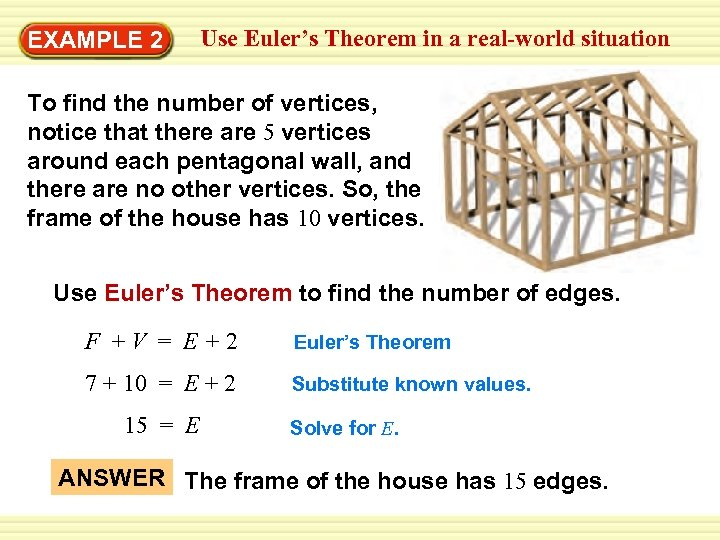Use Euler’s Theorem in a real-world situation Warm-Up EXAMPLE 2 Exercises To find the number of vertices, notice that there are 5 vertices around each pentagonal wall, and there are no other vertices. So, the frame of the house has 10 vertices. Use Euler’s Theorem to find the number of edges. F +V = E+2 Euler’s Theorem 7 + 10 = E + 2 Substitute known values. 15 = E Solve for E. ANSWER The frame of the house has 15 edges.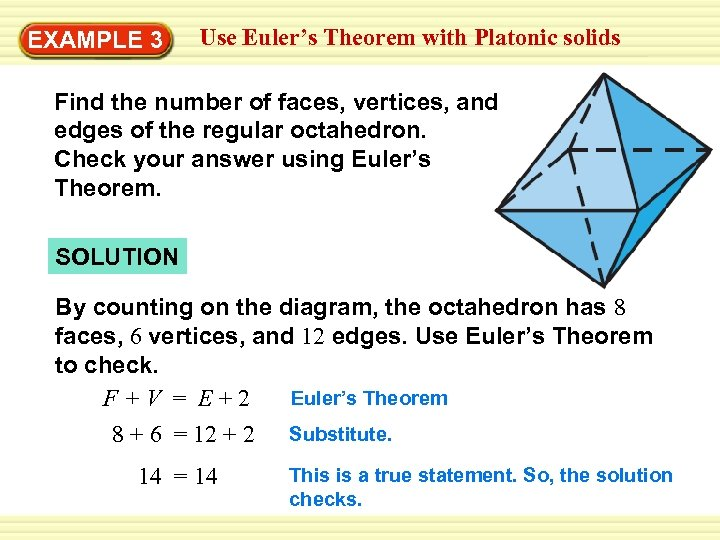Use Euler’s Theorem with Platonic solids Warm-Up EXAMPLE 3 Exercises Find the number of faces, vertices, and edges of the regular octahedron. Check your answer using Euler’s Theorem. SOLUTION By counting on the diagram, the octahedron has 8 faces, 6 vertices, and 12 edges. Use Euler’s Theorem to check. Euler’s Theorem F+V = E+2 8 + 6 = 12 + 2 Substitute. 14 = 14 This is a true statement. So, the solution checks.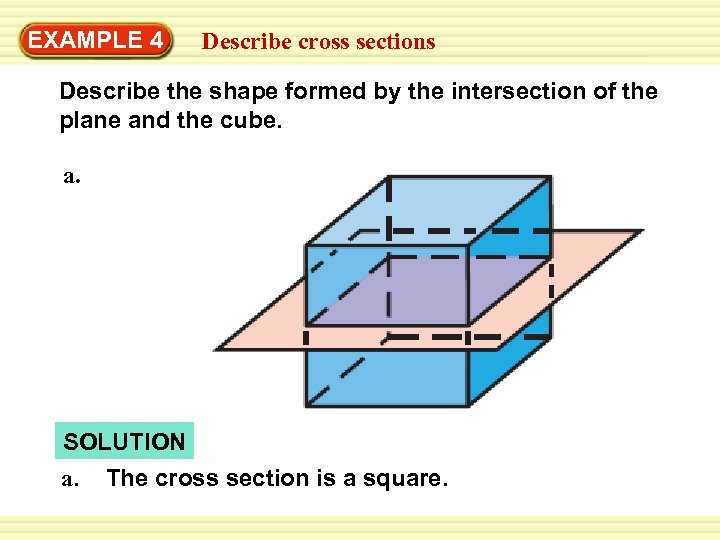Warm-Up EXAMPLE 4 Exercises cross sections Describe the shape formed by the intersection of the plane and the cube. a. SOLUTION a. The cross section is a square.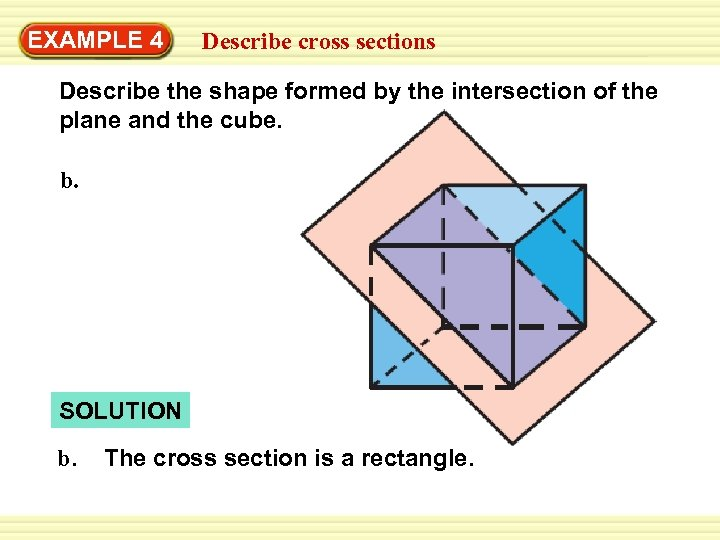Warm-Up EXAMPLE 4 Exercises cross sections Describe the shape formed by the intersection of the plane and the cube. b. SOLUTION b. The cross section is a rectangle.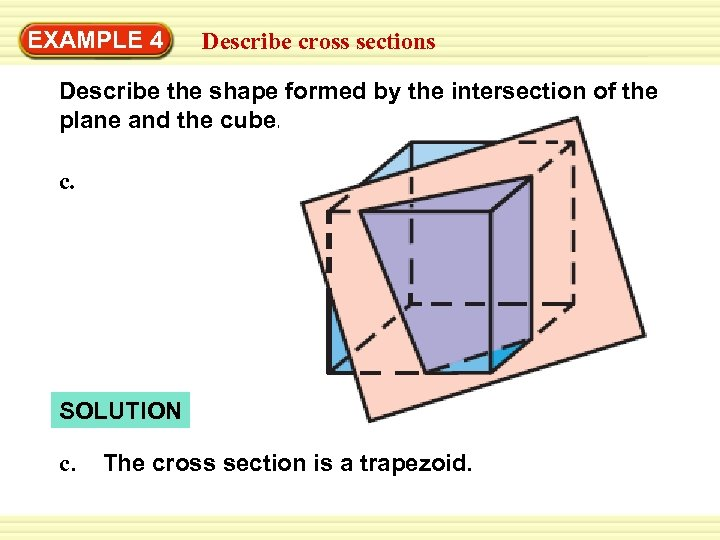Warm-Up EXAMPLE 4 Exercises cross sections Describe the shape formed by the intersection of the plane and the cube. c. SOLUTION c. The cross section is a trapezoid.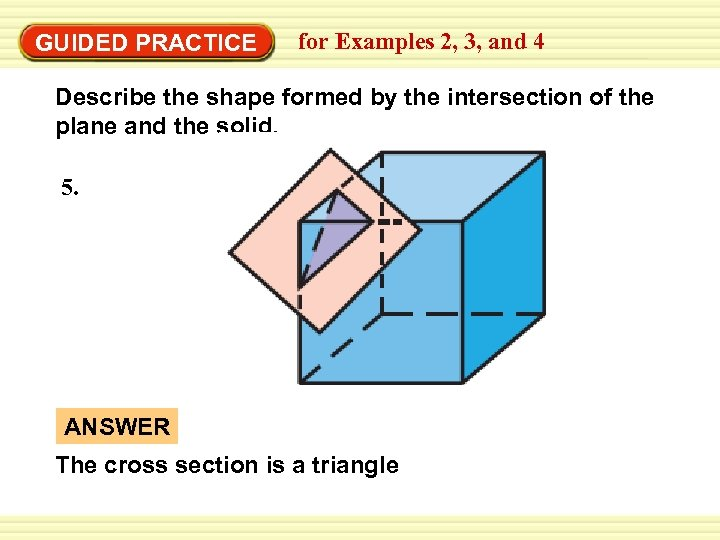Warm-Up Exercises GUIDED PRACTICE for Examples 2, 3, and 4 Describe the shape formed by the intersection of the plane and the solid. 5. ANSWER The cross section is a triangle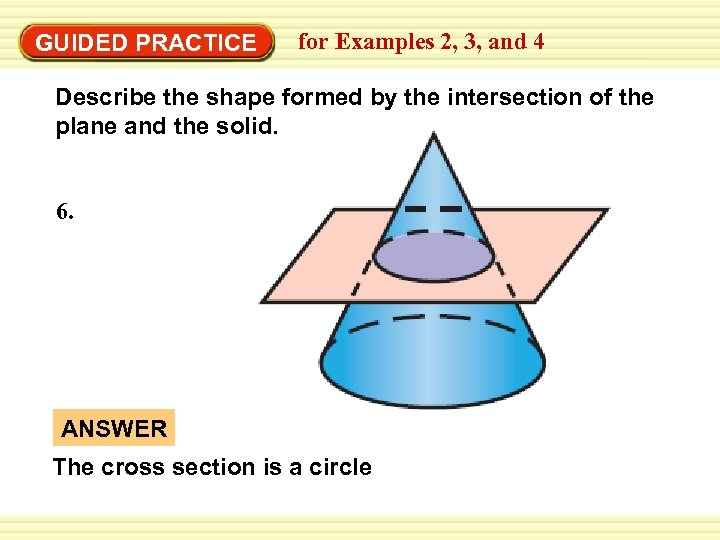Warm-Up Exercises GUIDED PRACTICE for Examples 2, 3, and 4 Describe the shape formed by the intersection of the plane and the solid. 6. ANSWER The cross section is a circle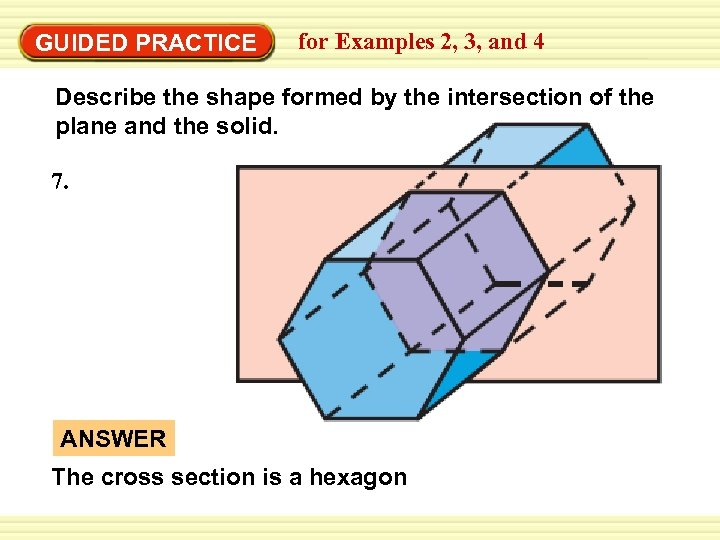Warm-Up Exercises GUIDED PRACTICE for Examples 2, 3, and 4 Describe the shape formed by the intersection of the plane and the solid. 7. ANSWER The cross section is a hexagon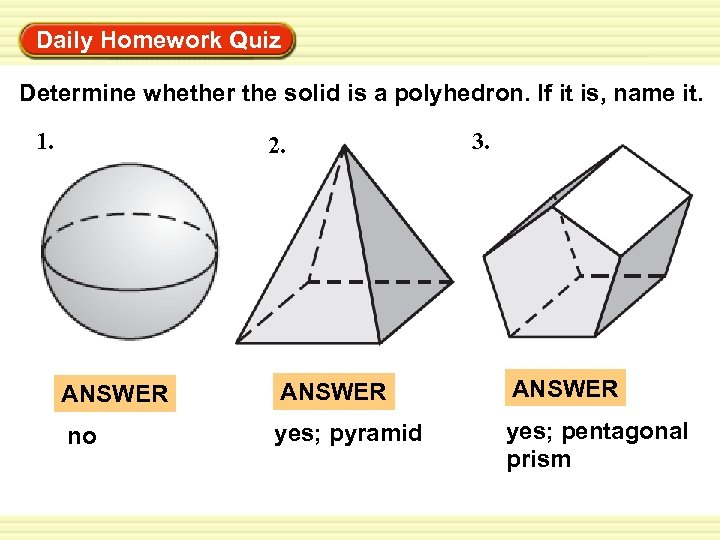Warm-Up Exercises Daily Homework Quiz Determine whether the solid is a polyhedron. If it is, name it. 1. 2. 3. ANSWER no yes; pyramid yes; pentagonal prism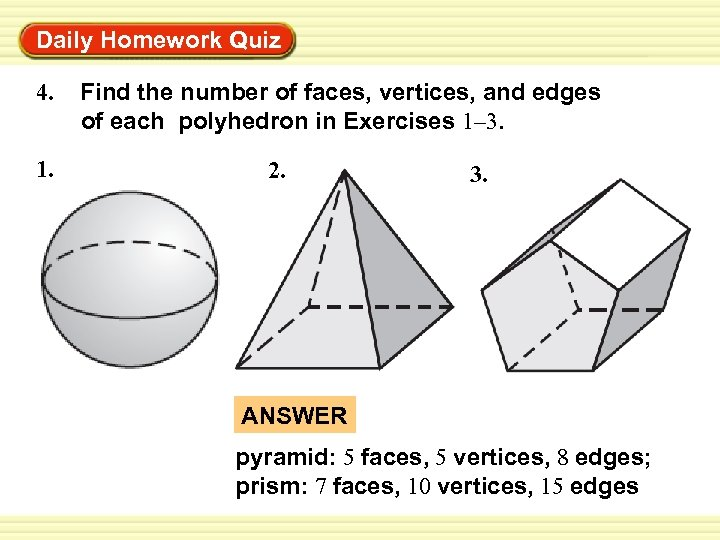Warm-Up Exercises Daily Homework Quiz 4. 1. Find the number of faces, vertices, and edges of each polyhedron in Exercises 1– 3. 2. 3. ANSWER pyramid: 5 faces, 5 vertices, 8 edges; prism: 7 faces, 10 vertices, 15 edges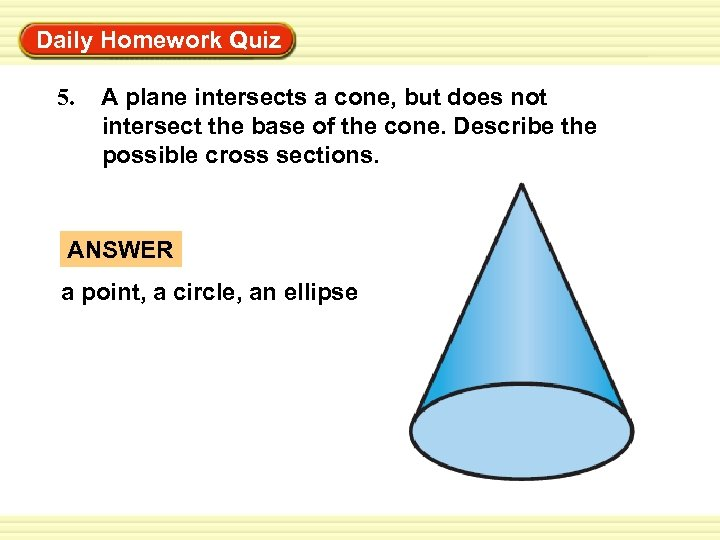Warm-Up Exercises Daily Homework Quiz 5. A plane intersects a cone, but does not intersect the base of the cone. Describe the possible cross sections. ANSWER a point, a circle, an ellipse